# Volcano plot in Python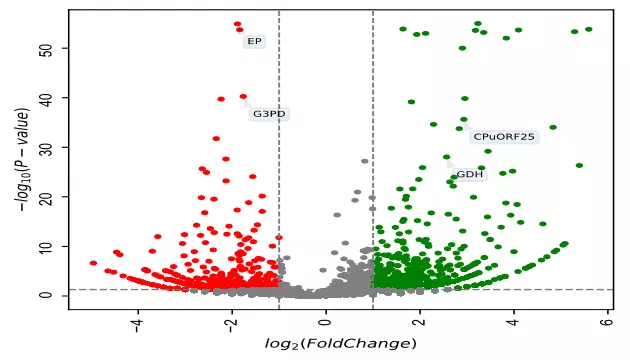## What is Volcano plot?

• Volcano plot is a 2-dimensional (2D) scatter plot having a shape like a volcano.
• Volcano plot used for visualization and identification of statistically significant gene expression changes from two different experimental conditions (e.g. normal vs. treated) in terms of log fold change (X-axis) and negative log10 of p value (Y-axis). The negative log 10 of p value used for scaling purposes which makes genes with lower p values appear at top of volcano plot. The higher the point on Y-axis, the lower is the p value.
• The wider dispersion of data points in the volcano plot indicates significant changes in gene expression between the two conditions.

## Applications

• Easy to visualize the expression of thousands of genes obtained from omics research (eg. Transcriptomics, genomics, proteomics, etc.) and pinpoint genes with significant changes

## How to create Volcano plot in Python?

• We will use `bioinfokit v2.0.8` or later
• Check bioinfokit documentation for installation and documentation (check how to install Python packages)
• For generating a volcano plot, I have used gene expression data published in Bedre et al. 2016 to identify statistically significantly induced or downregulated genes in response to salt stress in Spartina alterniflora (Read paper). Here’s you can download gene expression dataset used for generating volcano plot: dataset

Note: If you have your own dataset, you should import it as pandas dataframe. Learn how to import data using pandas

``````from bioinfokit import analys, visuz
# load dataset as pandas dataframe
df = analys.get_data('volcano').data
GeneNames  value1  value2    log2FC       p-value
0  LOC_Os09g01000.1    8862   32767 -1.886539  1.250000e-55
1  LOC_Os12g42876.1    1099     117  3.231611  1.050000e-55

visuz.GeneExpression.volcano(df=df, lfc='log2FC', pv='p-value')
# plot will be saved in same directory (volcano.png)
# set parameter show=True, if you want view the image instead of saving
``````

Generated volcano plot by above code (green: upregulated and red: downregulated genes),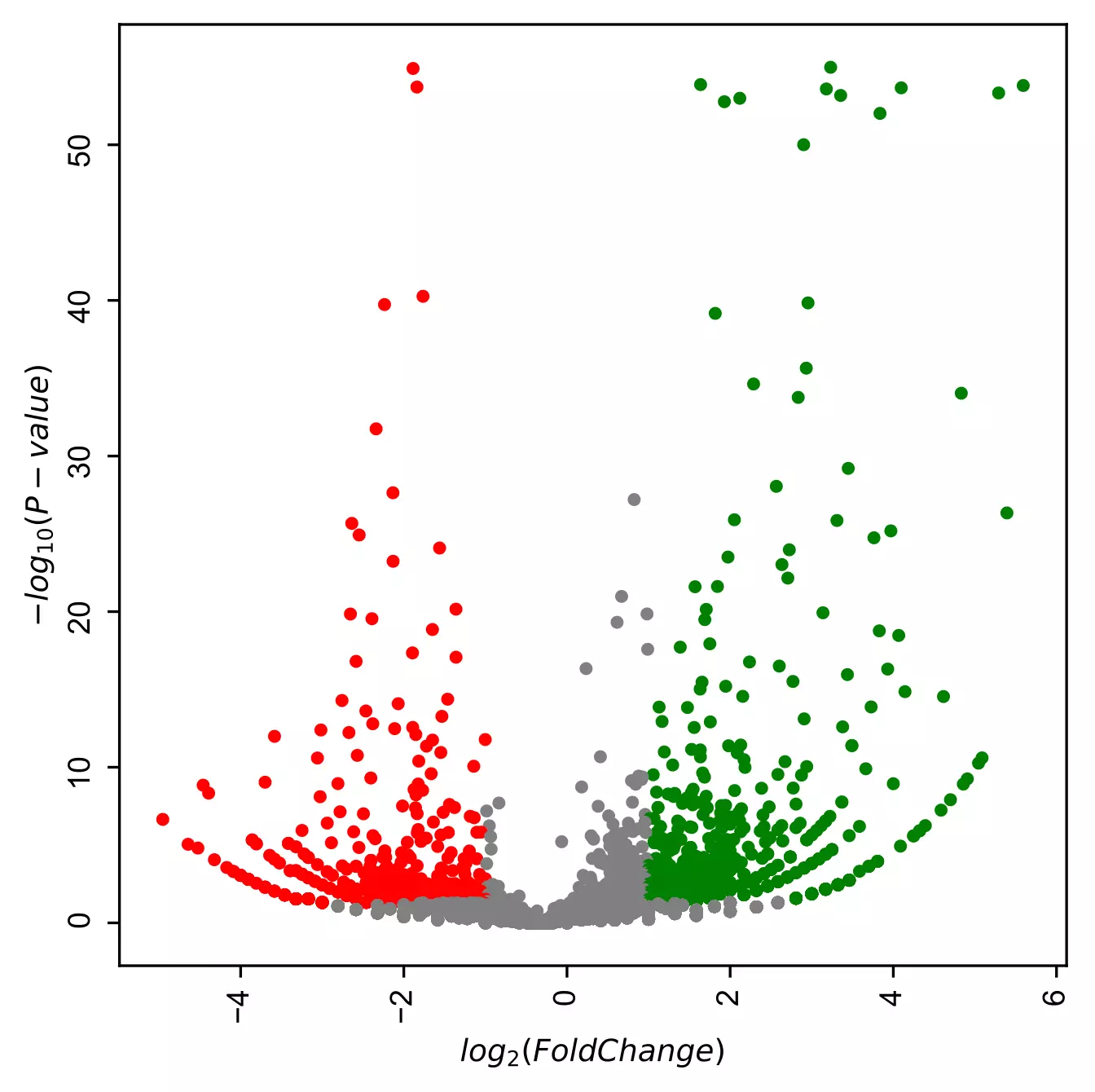Change background theme to dark,

``````visuz.GeneExpression.volcano(df=df, lfc='log2FC', pv='p-value', theme='dark')
``````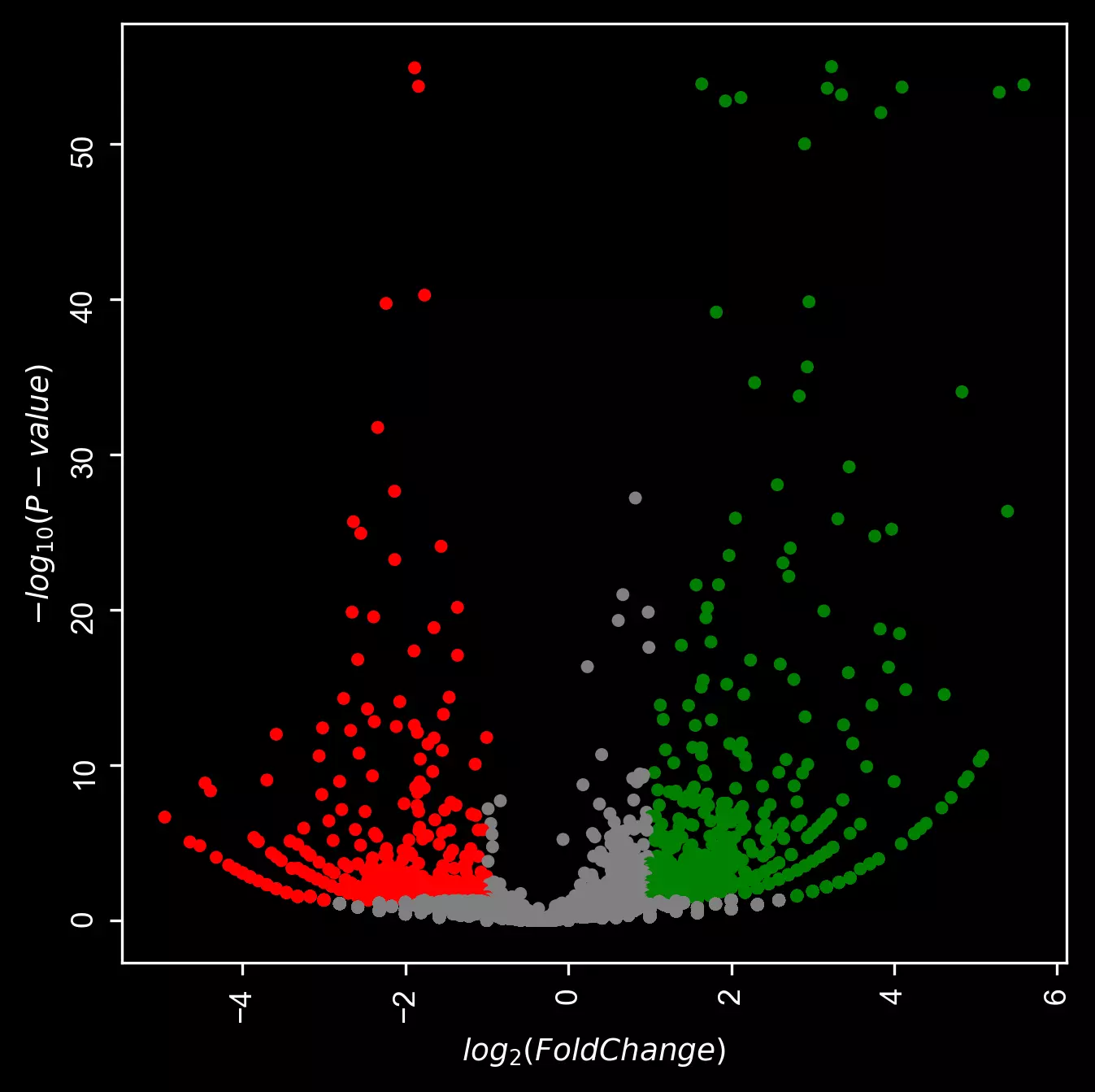``````visuz.GeneExpression.volcano(df=df, lfc='log2FC', pv='p-value', plotlegend=True, legendpos='upper right',
legendanchor=(1.46,1))
``````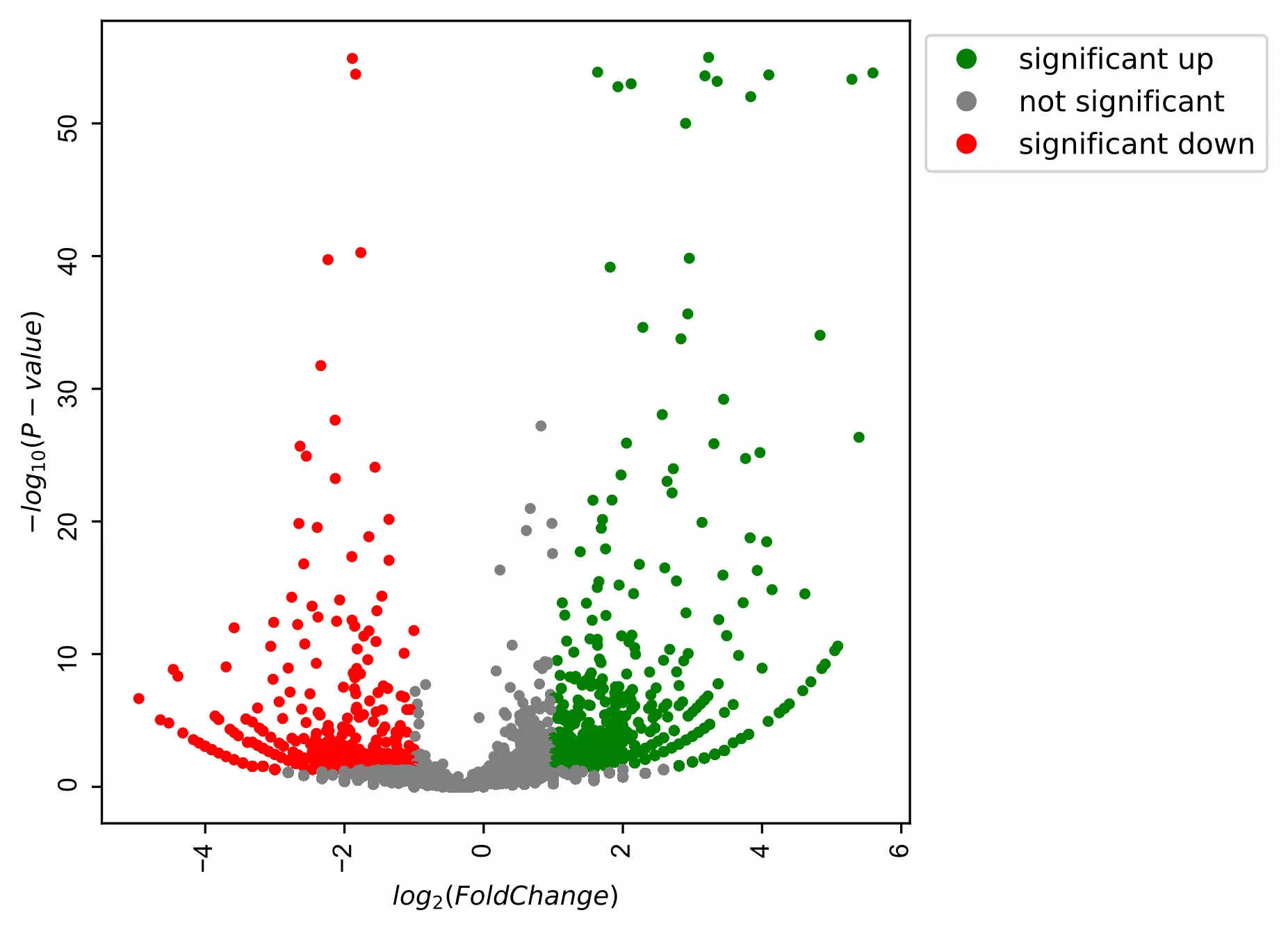Change color of volcano plot

``````# change colormap
visuz.GeneExpression.volcano(df=df, lfc='log2FC', pv='p-value', color=("#00239CFF", "grey", "#E10600FF"))
``````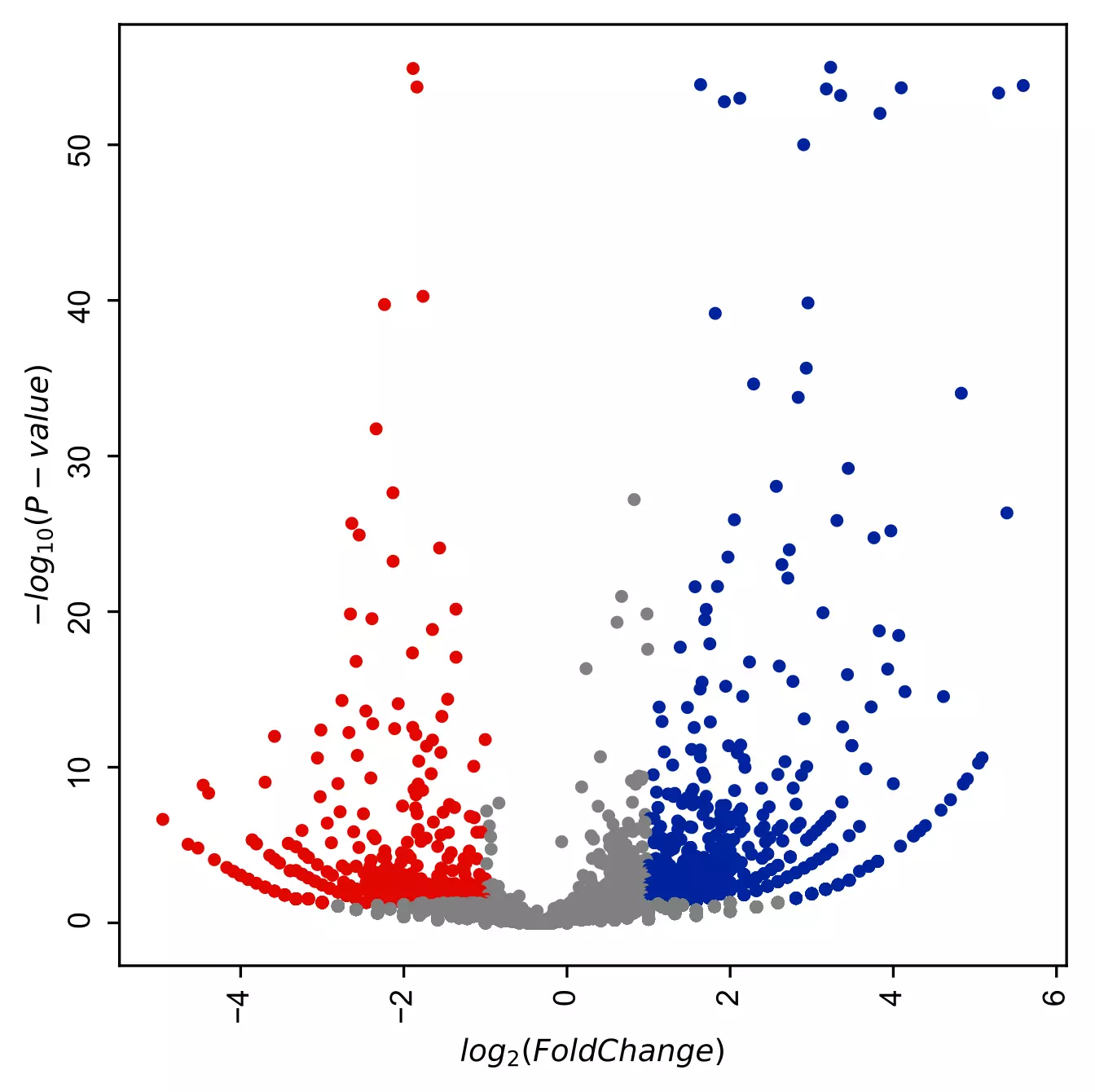Change log fold change and p value threshold,

``````visuz.GeneExpression.volcano(df=df, lfc='log2FC', pv='p-value', lfc_thr=(1, 2), pv_thr=(0.05, 0.01),
color=("#00239CFF", "grey", "#E10600FF"), plotlegend=True, legendpos='upper right',
legendanchor=(1.46,1))
``````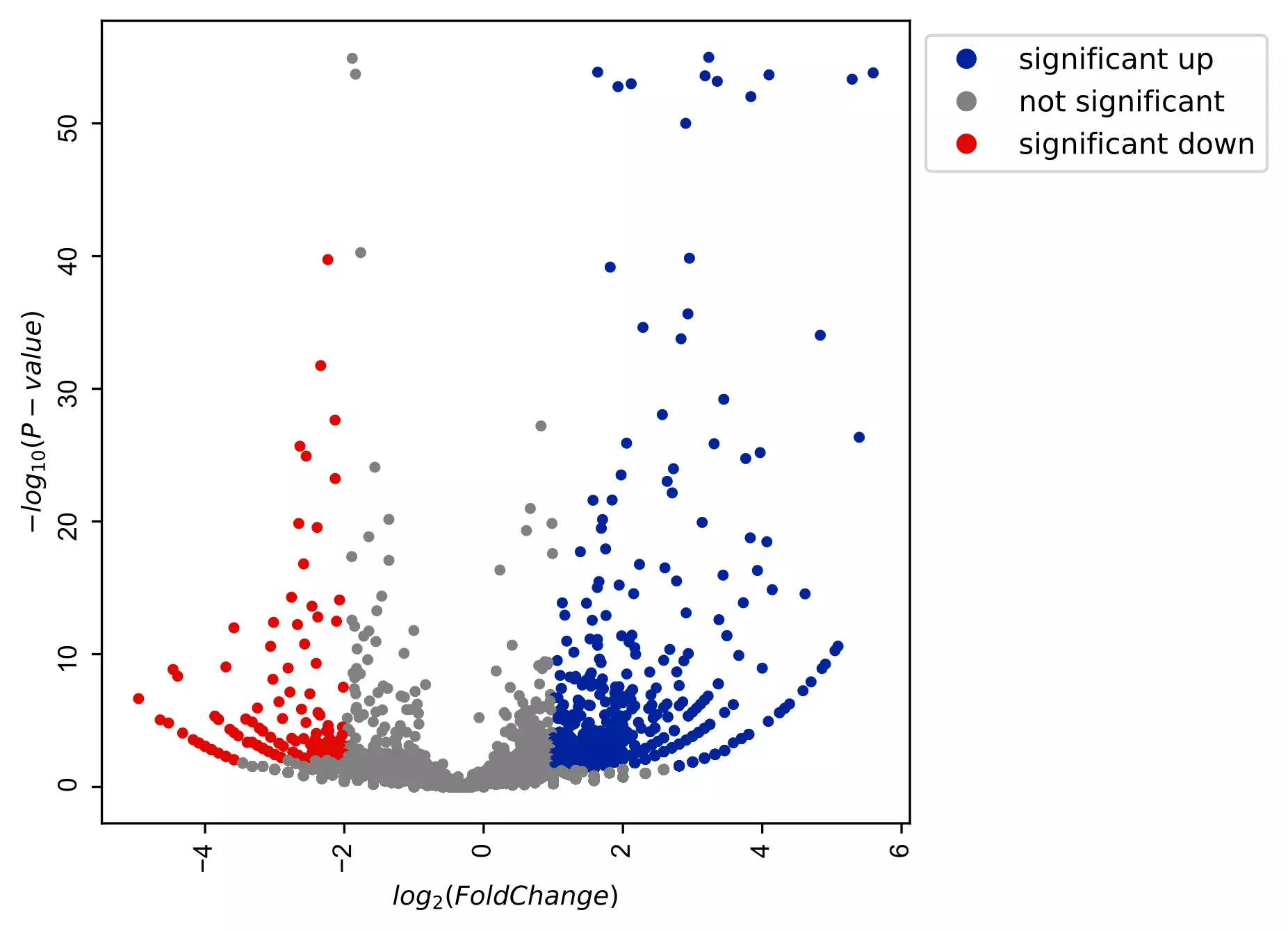Change transparency of volcano plot

``````visuz.GeneExpression.volcano(df=df, lfc='log2FC', pv='p-value', color=("#00239CFF", "grey", "#E10600FF"),
valpha=0.5)
``````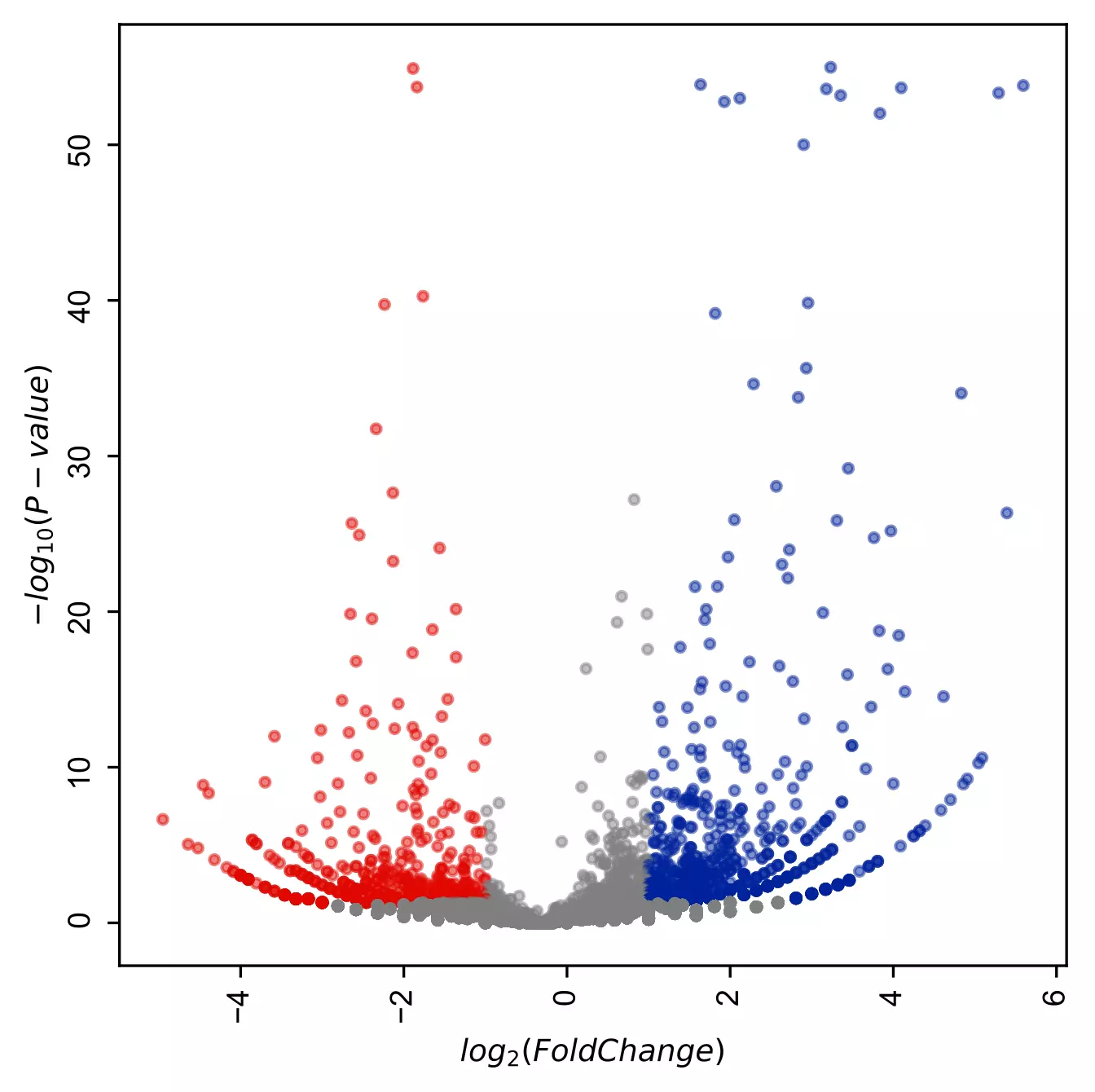Change the shape of the points

``````# add star shape
# check more shapes at https://matplotlib.org/3.1.1/api/markers_api.html
visuz.GeneExpression.volcano(df=df, lfc='log2FC', pv='p-value', color=("#00239CFF", "grey", "#E10600FF"),
markerdot='*')
``````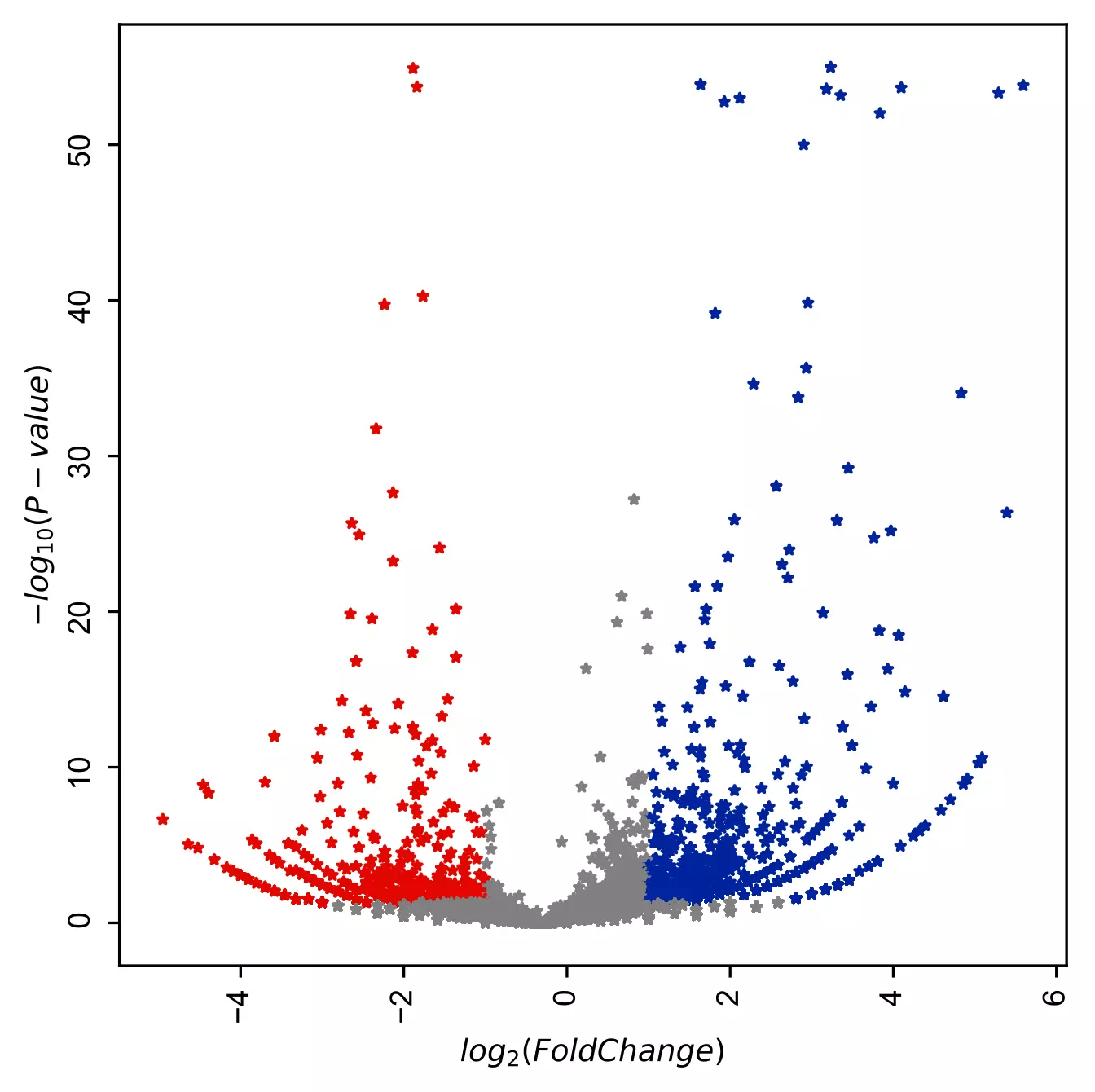Change the shape and size of the points

``````visuz.GeneExpression.volcano(df=df, lfc='log2FC', pv='p-value', color=("#00239CFF", "grey", "#E10600FF"),
markerdot='*', dotsize=30)
``````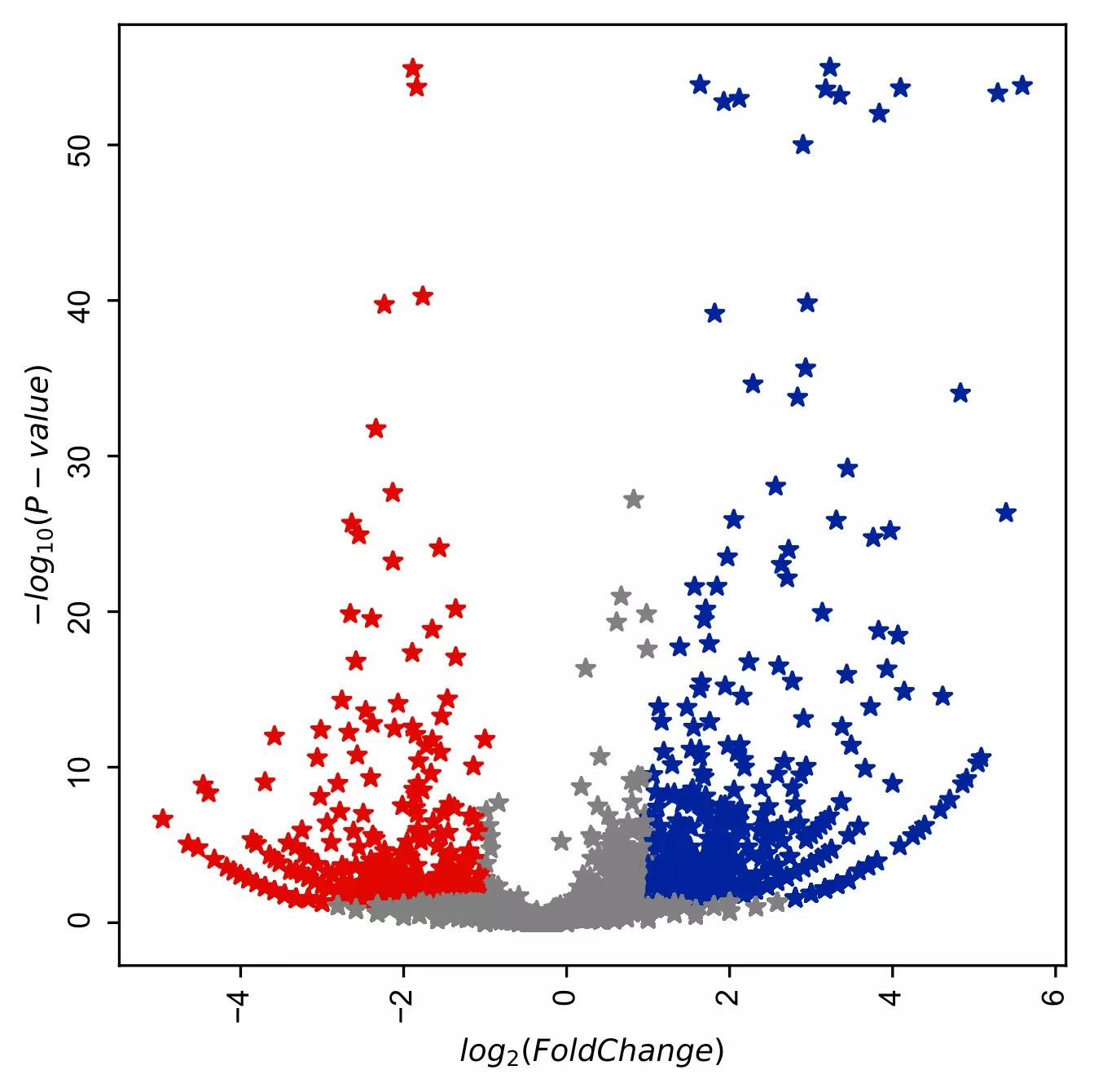Add gene labels (text style) to the points,

``````# add gene customized labels
# note: here you need to provide column name of gene Ids (geneid parameter)
# default simple text will be added
visuz.GeneExpression.volcano(df=df, lfc="log2FC", pv="p-value", geneid="GeneNames",
genenames=("LOC_Os09g01000.1", "LOC_Os01g50030.1", "LOC_Os06g40940.3", "LOC_Os03g03720.1") )
# if you want to label all DEGs defined lfc_thr and pv_thr, set genenames='deg'
``````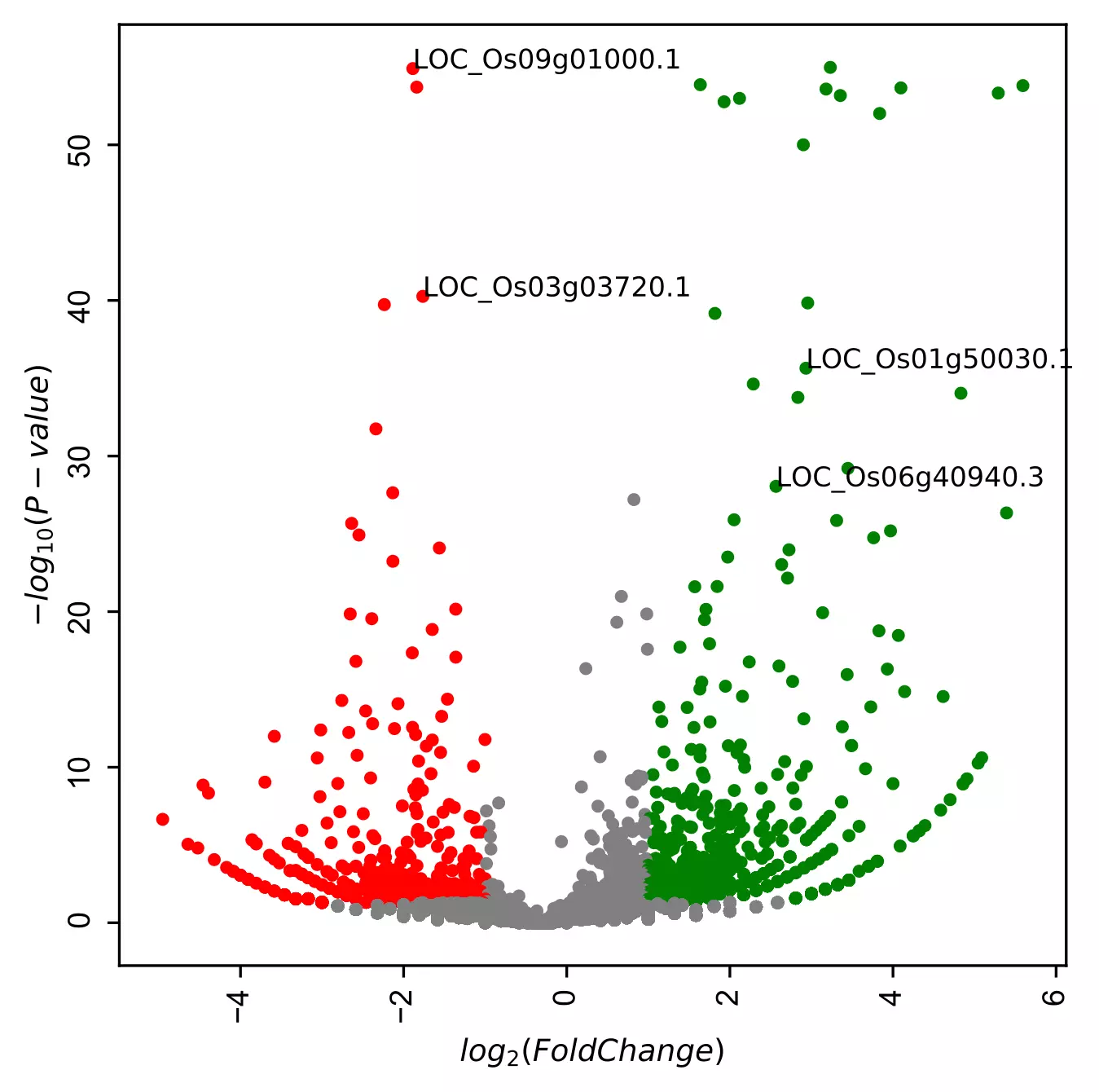Add gene labels (box style) to the points,

``````visuz.GeneExpression.volcano(df=df, lfc="log2FC", pv="p-value", geneid="GeneNames",
genenames=("LOC_Os09g01000.1", "LOC_Os01g50030.1", "LOC_Os06g40940.3", "LOC_Os03g03720.1"), gstyle=2 )
``````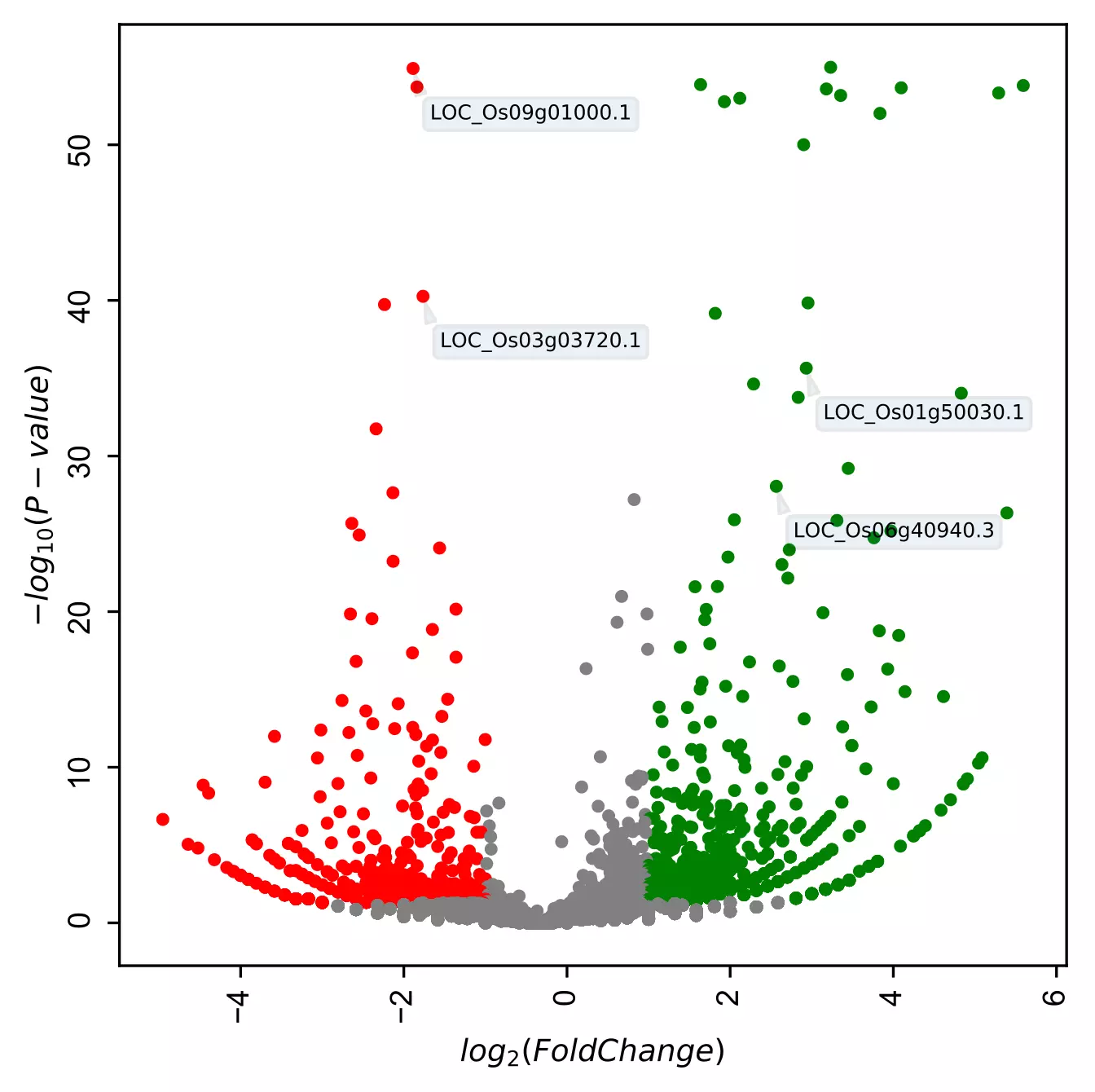``````# add gene customized labels
# note: here you need to provide column name of gene Ids (geneid parameter)
# as the dataset only have geneids, you need to provide tuple of gene Id and corresponding gene names
visuz.GeneExpression.volcano(df=df, lfc="log2FC", pv="p-value", geneid="GeneNames",
genenames=({"LOC_Os09g01000.1":"EP", "LOC_Os01g50030.1":"CPuORF25", "LOC_Os06g40940.3":"GDH", "LOC_Os03g03720.1":"G3PD"}),
gstyle=2)
``````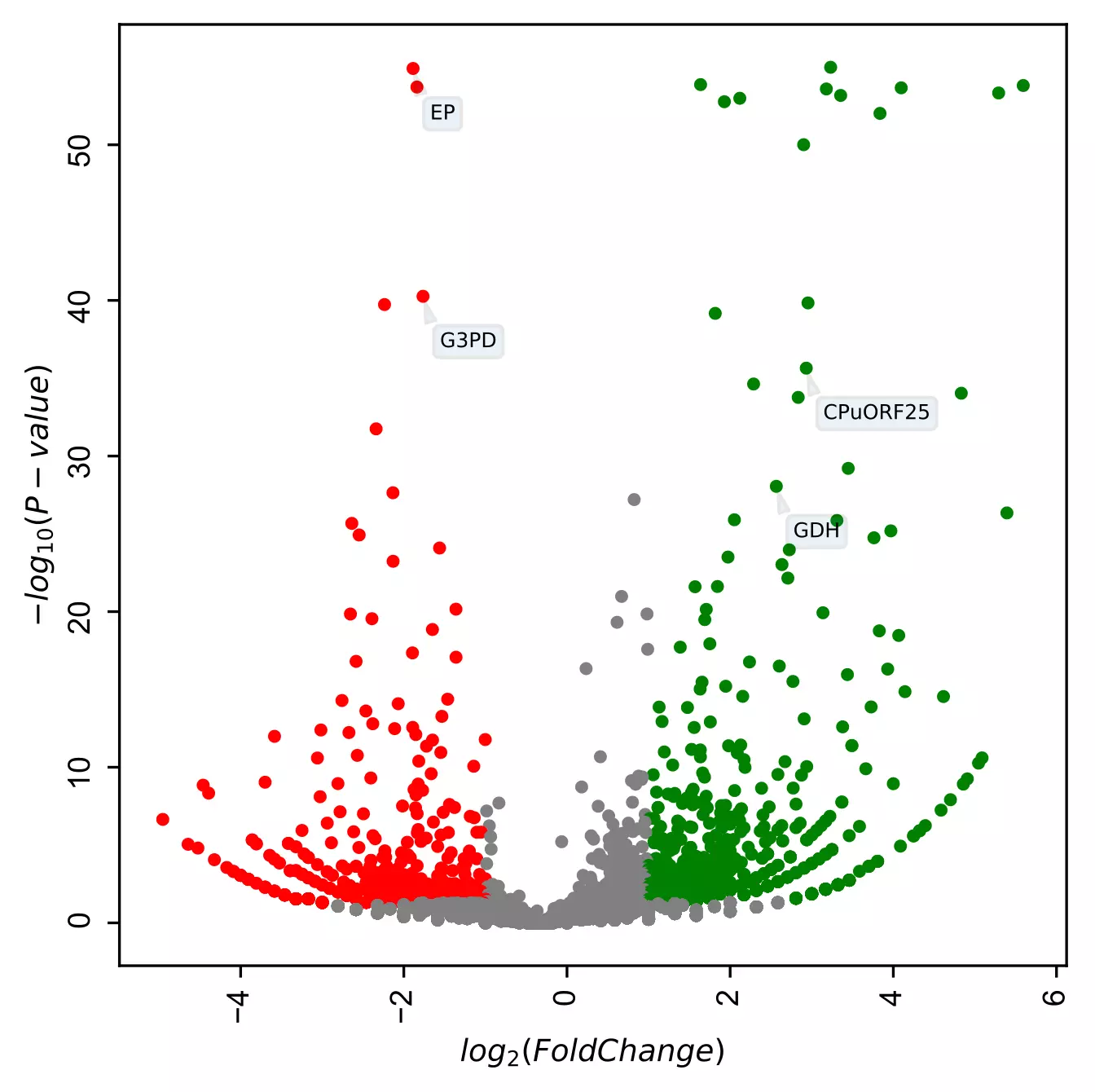``````visuz.GeneExpression.volcano(df=df, lfc="log2FC", pv="p-value", geneid="GeneNames",
genenames=({"LOC_Os09g01000.1":"EP", "LOC_Os01g50030.1":"CPuORF25", "LOC_Os06g40940.3":"GDH", "LOC_Os03g03720.1":"G3PD"}),
gstyle=2, sign_line=True)
``````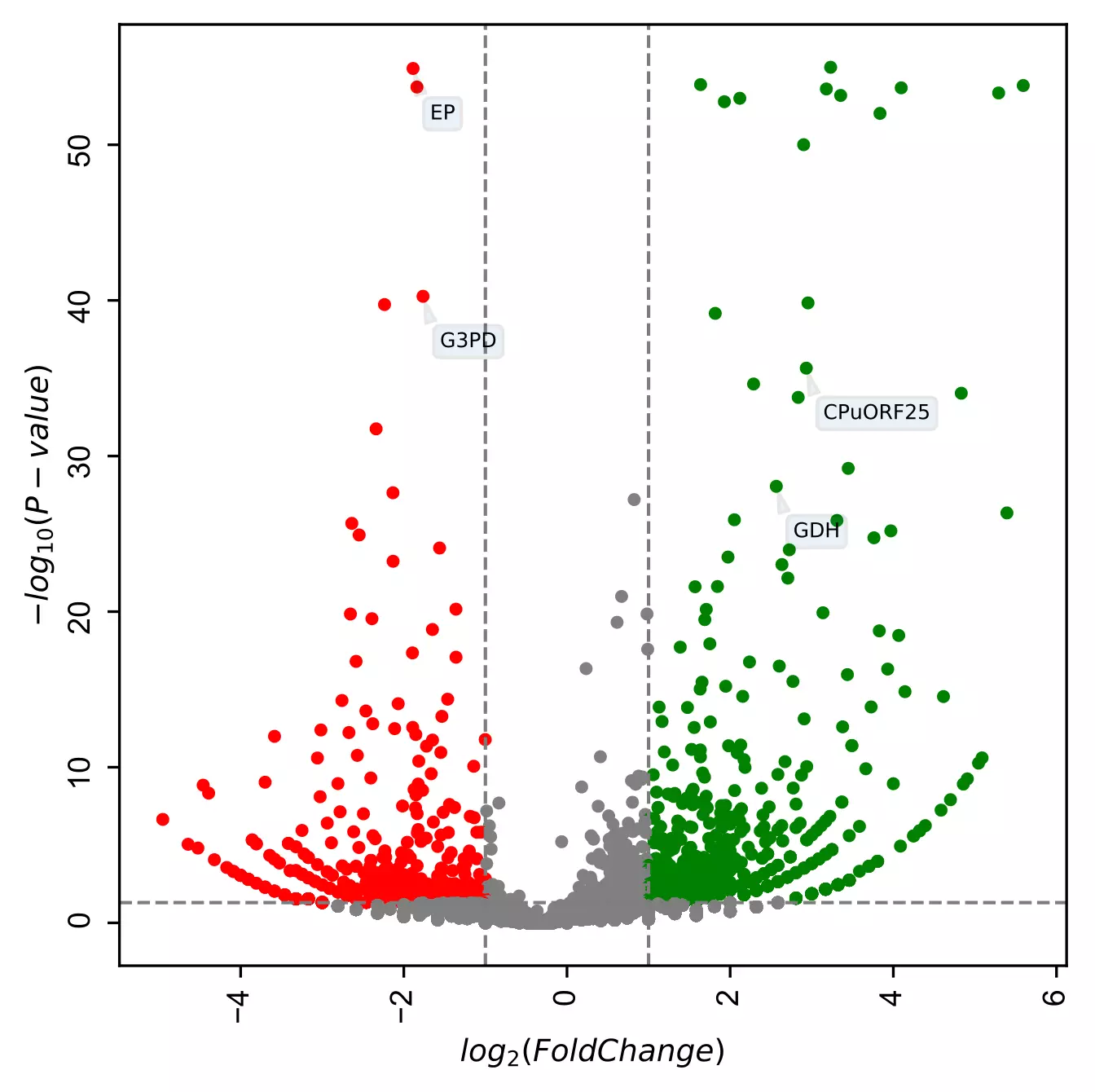Change X and Y range ticks, font size and name for tick labels

``````visuz.GeneExpression.volcano(df=df, lfc="log2FC", pv="p-value", geneid="GeneNames",
genenames=({"LOC_Os09g01000.1":"EP", "LOC_Os01g50030.1":"CPuORF25", "LOC_Os06g40940.3":"GDH", "LOC_Os03g03720.1":"G3PD"}),
gstyle=2, sign_line=True, xlm=(-6,6,1), ylm=(0,61,5), figtype='svg', axtickfontsize=10,
axtickfontname='Verdana')
``````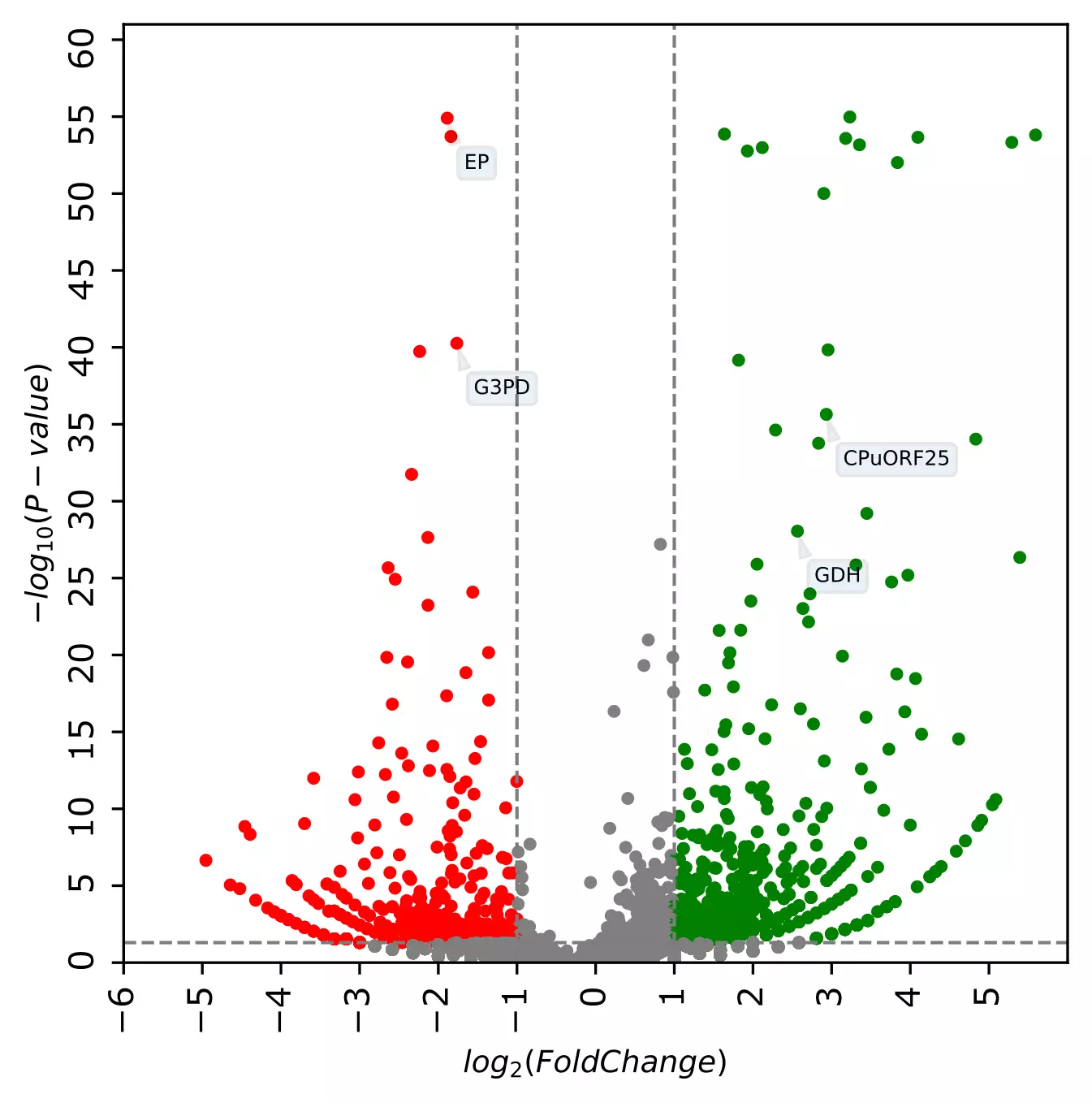In addition to these parameters, the parameters for figure type (`figtype`), X and Y axis ticks range (`xlm`, `ylm`), axis labels (`axxlabel`, `axylabel`),
axis labels font size (`axlabelfontsize`), and axis tick labels font size and name (`axtickfontsize`, `axtickfontname`) can be provided.

To create a inverted volcano plot,

``````# you can use interactive python console, jupyter or python code
# I am using interactive python console
from bioinfokit import visuz
# here you can change default parameters.
visuz.GeneExpression.involcano(df=df, lfc="log2FC", pv="p-value")
``````

Generated inverted volcano plot by adding above code,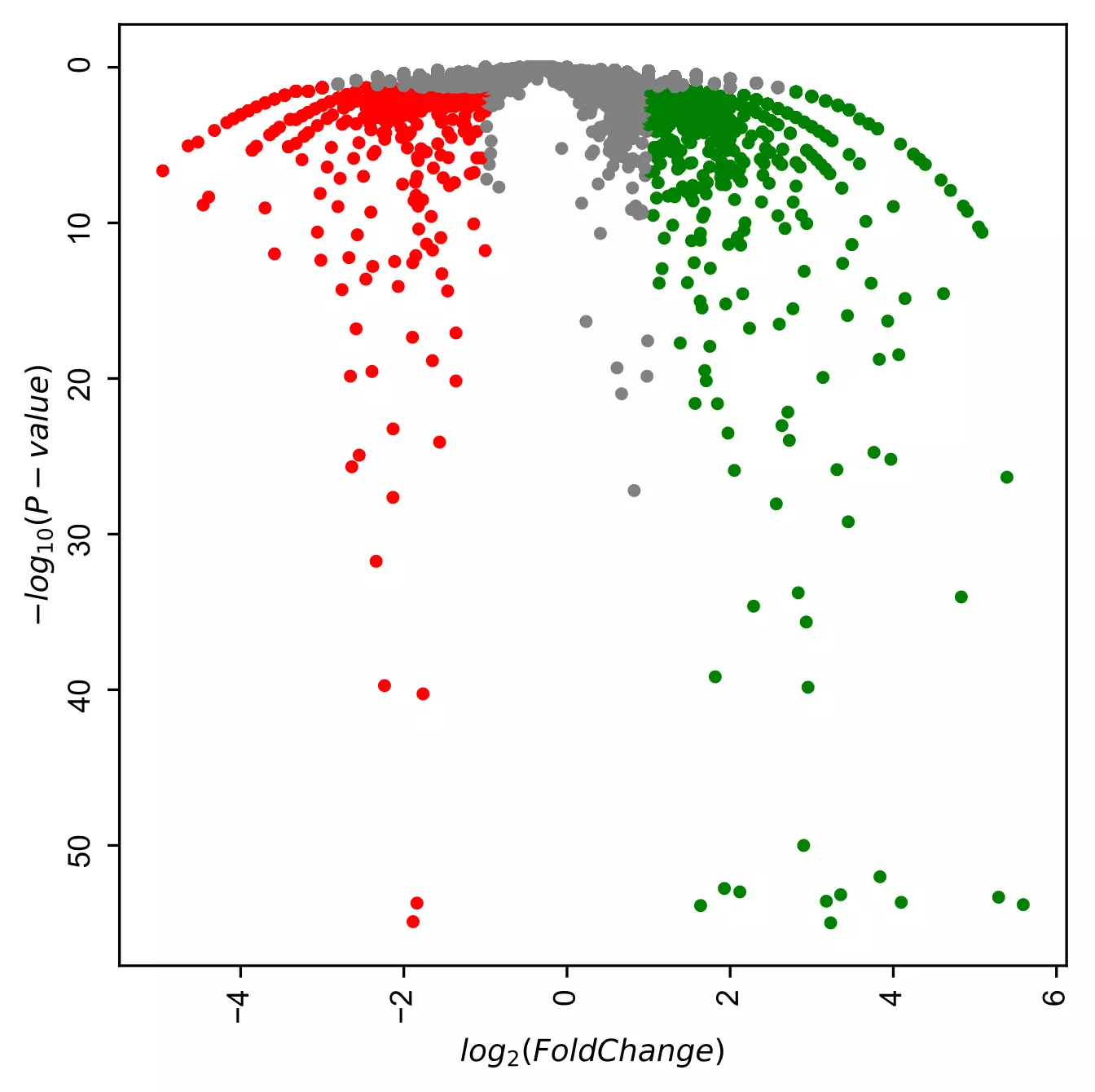Change color inverted volcano plot

``````# change colormap
visuz.GeneExpression.involcano(df=df, lfc="log2FC", pv="p-value", color=("#00239CFF", "grey", "#E10600FF"))
``````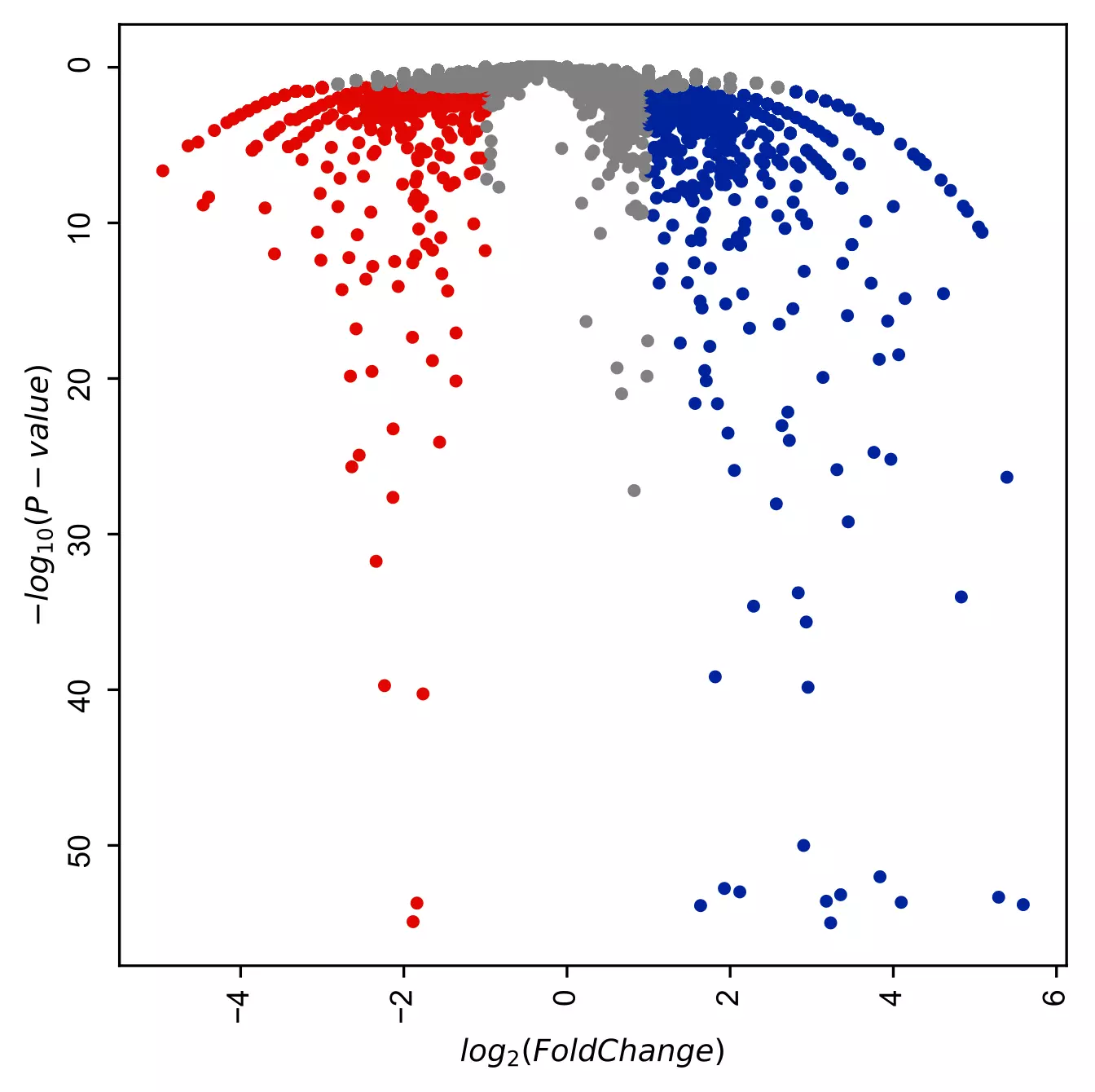``````# add gene customized labels
# note: here you need to provide column name of gene Ids (geneid parameter)
# as the dataset only have geneids, you need to provide tuple of gene Id and corresponding gene names
visuz.GeneExpression.involcano(df=df, lfc="log2FC", pv="p-value", geneid="GeneNames",
genenames=({"LOC_Os09g01000.1":"EP", "LOC_Os01g50030.1":"CPuORF25", "LOC_Os06g40940.3":"GDH", "LOC_Os03g03720.1":"G3PD"}),
gstyle=2)
``````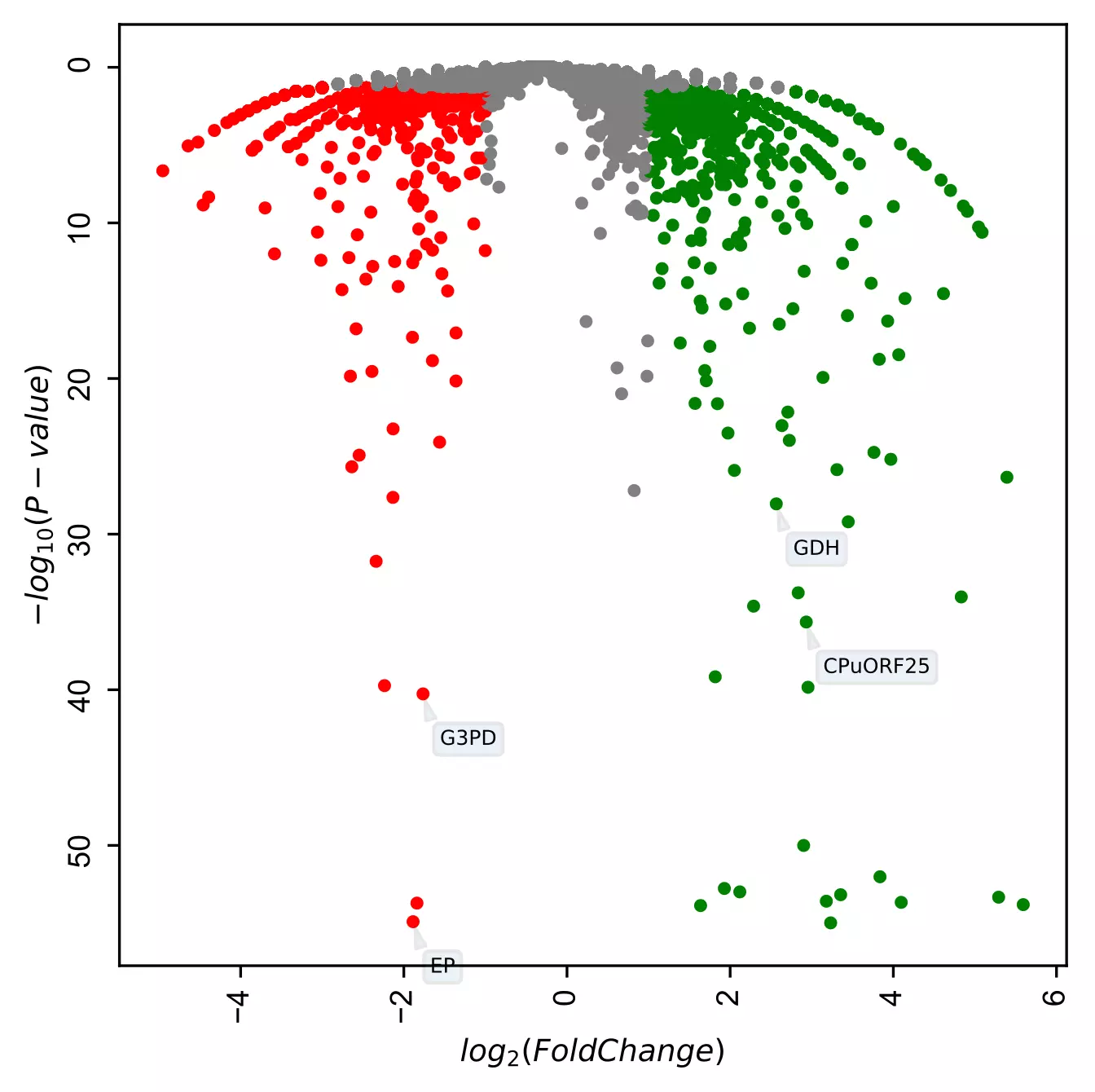In addition to these parameters, the parameters for figure type (`figtype`), axis labels (`axxlabel`, `axylabel`), axis labels font size (`axlabelfontsize`), and axis tick labels font size and name (`axtickfontsize`, `axtickfontname`) can be provided.

If you have any questions, comments or recommendations, please email me at reneshbe@gmail.com